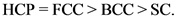# Rank the crystal lattice structures in order of decreasing efficiency of space in the structure

Rank the crystal lattice structures in order of decreasingefficiency of space in the structure.
1.Simple cubic
2.Body centered cubic
3.Face centered cubic
4.Hexegonal close packed
I know simple cubic is the least effcient, and i figured itwhould be HCP-FCC-BCC-Simple cubic,

Concepts and reason
Matter exists in various forms such as gaseous state, liquid state, and solid state. In solid state, the constituent particles are arranged very closely. Solids mainly exist in two forms, such as amorphous solids and crystalline solids.
Crystalline solid has rigidity, incompressibility, and specific shape. The crystalline solids are ordered by arrangement of atoms, ions, or molecules.

Fundamentals
There are seven major types of crystals depending on the shape of the crystal.

• Cubic
• Tetragonal
• Hexagonal
• Rhombohedral
• Orthorhombic
• Monoclinic
• Triclinic

In crystallography, the packing efficiency of crystals is calculated by atomic packing factor. This is defined as the fraction of volume or space occupied by atom in a unit cell.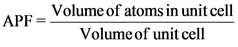The known values of the atomic packing factor or packing efficiency of crystal structure is as follows.

For simple cubic (SC):
The atomic packing factor for SC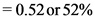For body-centered cubic (BCC):
The atomic packing factor for BCC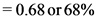For face-centered cubic (FCC):
The atomic packing factor for FCC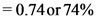For hexagonal close packing (HCP):
The atomic packing factor for HCPExplanation:
The atomic packing factor for simple cubic, body-centered cubic, face-centered cubic, and hexagonal close packing are mentioned above.

The fraction of free space or volume of SC: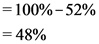The fraction of free space or volume of BCC: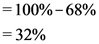The fraction of free space or volume of FCCThe fraction of free space or volume of HCPTherefore, the order of decreasing efficiency of space in the given structure is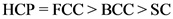Explanation:
The free space for simple cubic, body-centered cubic, face-centered cubic, and hexagonal close packing are calculated by subtraction of fraction value of crystal with 100% Therefore, order of decreasing efficiency of space in the given crystal structure is